One of the main problems of rendering is its perfection. Tree objects, that consist of many leaf elements with the same material applied to them, are often lack the natural variations the viewer would expect. In our production workflow we faced this problem on a daily basis. As we always try to improve the quality of our architectural renderings we decided to create an easy to use script to set random material IDs to give the perception of natural variations.

Our main content creation software is 3ds Max, so we created a Macro Script (in MAXScript) for this problem.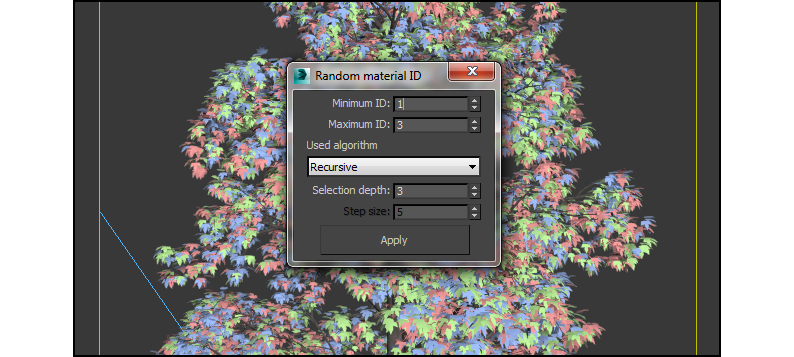Recursive or iterative approach

There are basically two possible approaches to handle this problem. The iterative first oneone iterates through all faces for setting a random material ID while the recursive one version divides the big vast amount of faces into smaller parts groups(groups?) and then assigns a material ID to all the elements contained in this group. ?.connected to the face selection.

At first we implemented the iterative approach to take a look at its possibilities and restrictions. After that we thought about possible optimizations and other ideas how to solve this problem more efficiently with an optimum balance of between speed and a visual qualitygood result. That’s why we created further looked into the a recursive version to solve the problem from another perspective point of view.

Algorithms

Iterative version

```function changeIDsIterative obj minID maxID stepSize =
(
getElement = polyop.getElementsUsingFace
setFaceID = polyop.setFaceMatID

range = 1

for i = 1 to obj.numfaces by stepSize do (

randomID = random minID maxID

range = i + stepSize

if(range > obj.numfaces) do (
range = obj.numfaces
)

element = getElement obj #{i..range}
setFaceID obj element randomID
)
)
```

Basically this script iterates through all faces with a given step. There it takes the takes the elements which are connected with the faces within the step range and gives this selection of faces a random material ID in the range of the minimal ID and the maximal ID (minID <= ID <= maxID).

Larger values of stepsizestep size would give result in more speed by skipping a lot of faces but would result in a visible repetition of the same used material ID.

So the number of function calls when to setting a random material ID depends on the step size s:You may have noticed that in the first two lines two function calls are assigned to variables.

```getElement = polyop.getElementsUsingFace
setFaceID = polyop.setFaceMatID
```

That’s because ofThis is done for performance reasons. By Iinitializing the function call as a variable these many function callswill allow it to run are slightly faster.

Recursive version

```function changeIDs depth obj minFace maxFace minID maxID =
(
if(depth > 0) then ( --Clustering, if depth != 0
partSize = ((maxFace - minFace) / 2 + 1) as Integer --calculate the clustersize

changeIDs (depth-1) obj minFace (partSize + minFace) minID maxID -- first part
changeIDs (depth-1) obj (partSize + minFace + 1) maxFace minID maxID -- second part

) else ( --set material IDs
getElement = polyop.getElementsUsingFace
setFaceID = polyop.setFaceMatID

randomID = random minID maxID
--get a random ID between the given values
element = getElement obj #{minFace..maxFace}
--select all elements with faces in the given cluster of faces
setFaceID obj element randomID
--set the random ID to all faces in the selection
)
)
```

The recursive version divides the huge amount of into smaller clusters of faces before setting a material ID.

The algorithm basically divides the amount of faces into halves until the depth value is zero. Then for every subpart the script selects all elements which are connected with the faces of the subpart and assigns a random ID. So the second part is equal to the iterative version.

The only difference is the manner of selecting the faces.

Example for depth = 3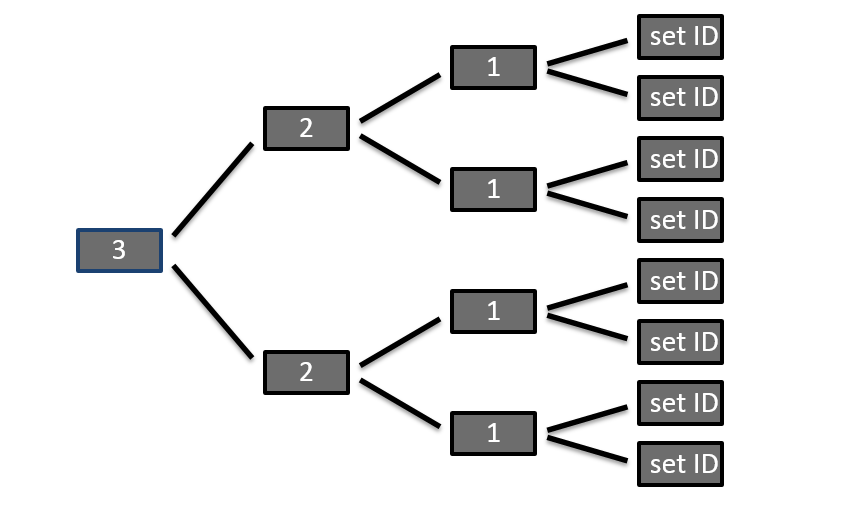So the number of parts where to set a random material ID is:Dividing the amount of faces recursively into subparts is very fast. The main bottleneck of this script is the access of the 3dx Max-Interfaces getElement and setFaceID.

The equivalent parameters for iterative and recursive function calls can be calculated by:Which one is better now?

Basically both version do the same work and should be able to give a comparable result. The main differences are the parameters and the internal procedure.

If you have an object with a lot of polygons and (nearly) every element should have a random material ID then the iterative version will be easier to use because you simply enter 1 or comparable low values for the step size s. or other small values. Then the script would go assignto a random ID to every face and set a random ID. But On the other hand if the elements have a lot of faces the work would often be done more than once. This will be also very slow because of many interface accesses of 3ds Max.

Then it would be easier to use the recursive approach and begin with small values for the depth until you get a satisfying result with enough mixture by increasing the depth value.

Examples

Different material IDs: 3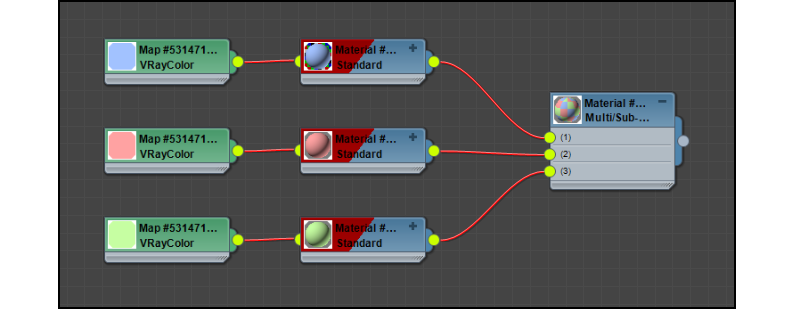Duration – iterative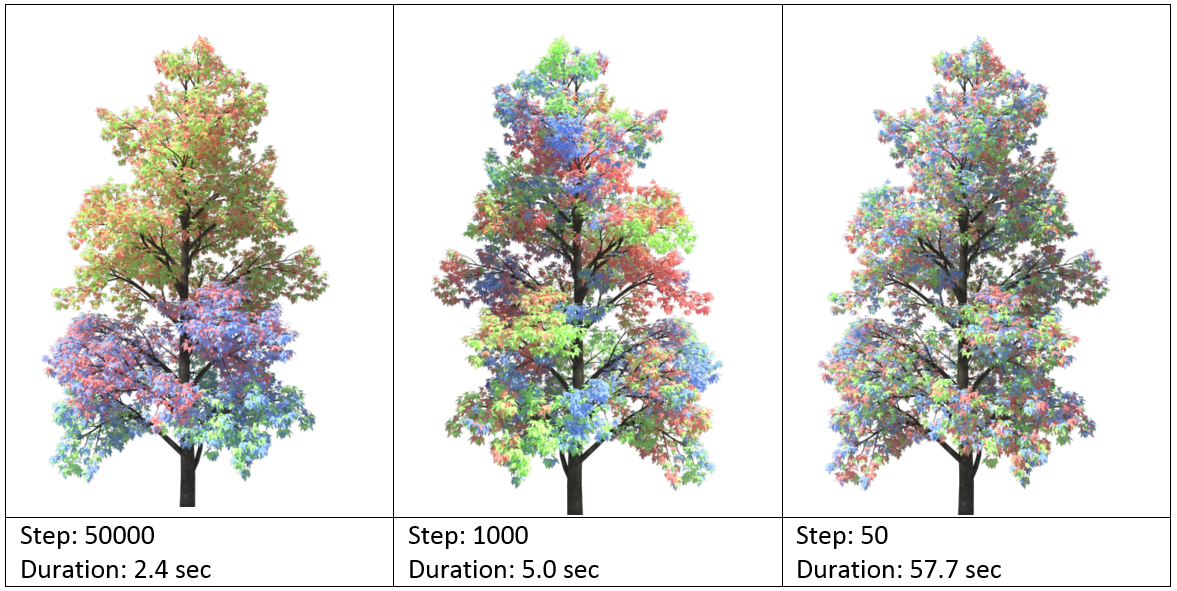Duration – recursive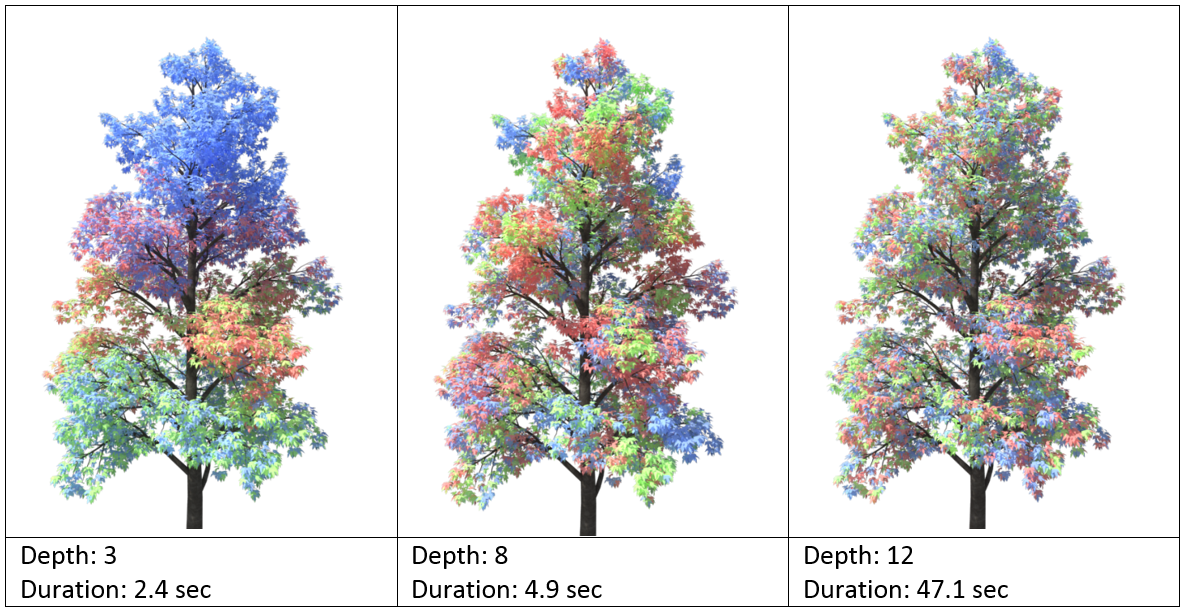It was a pleasure for us to show you these little thoughts about our workflow. We hope you had fun while reading the text and looking to the pictures. To see more of our projects, visit our Showroom.